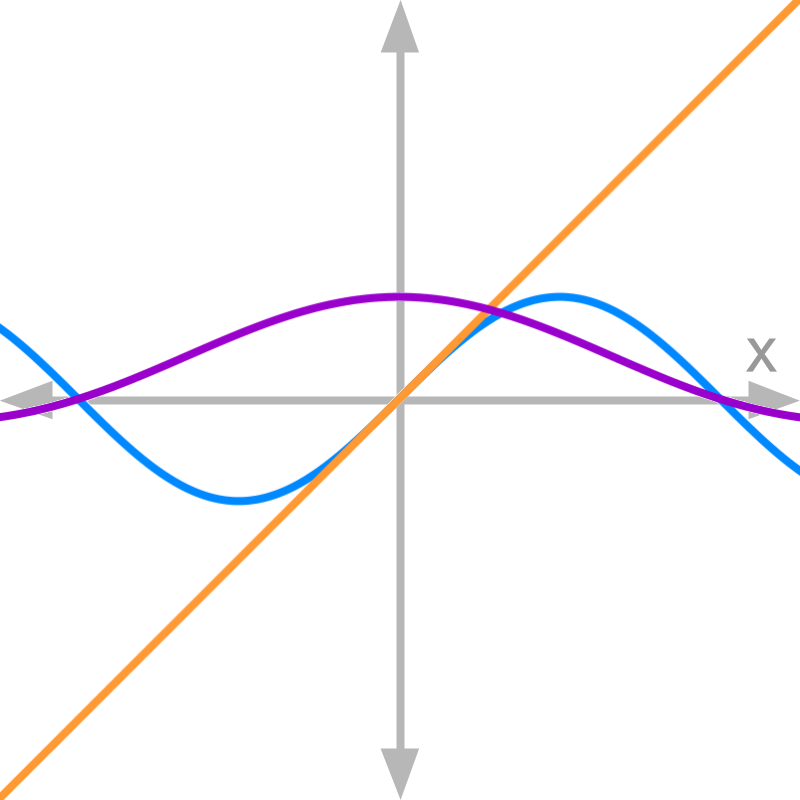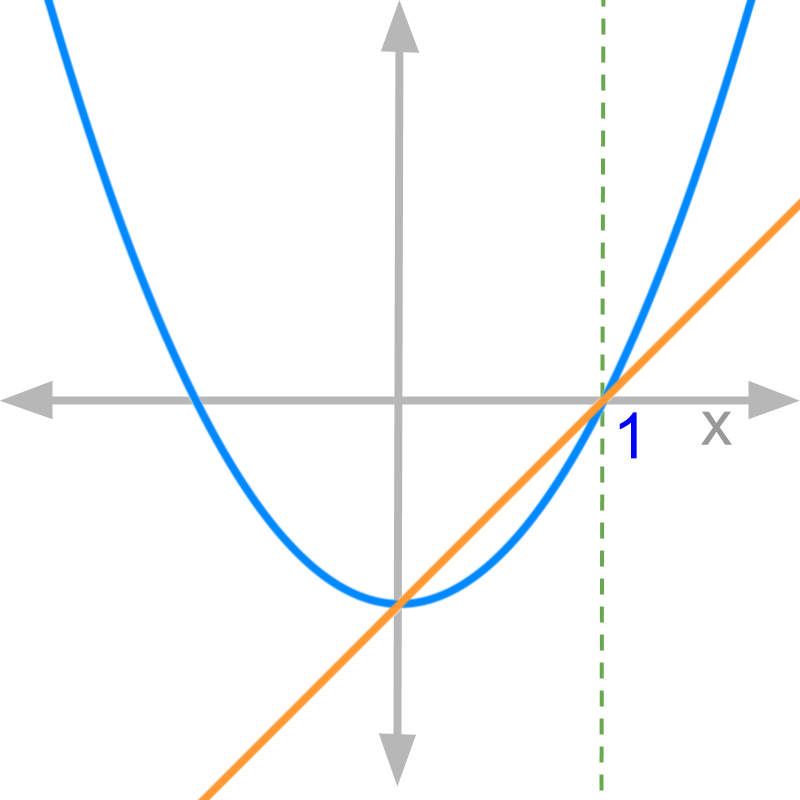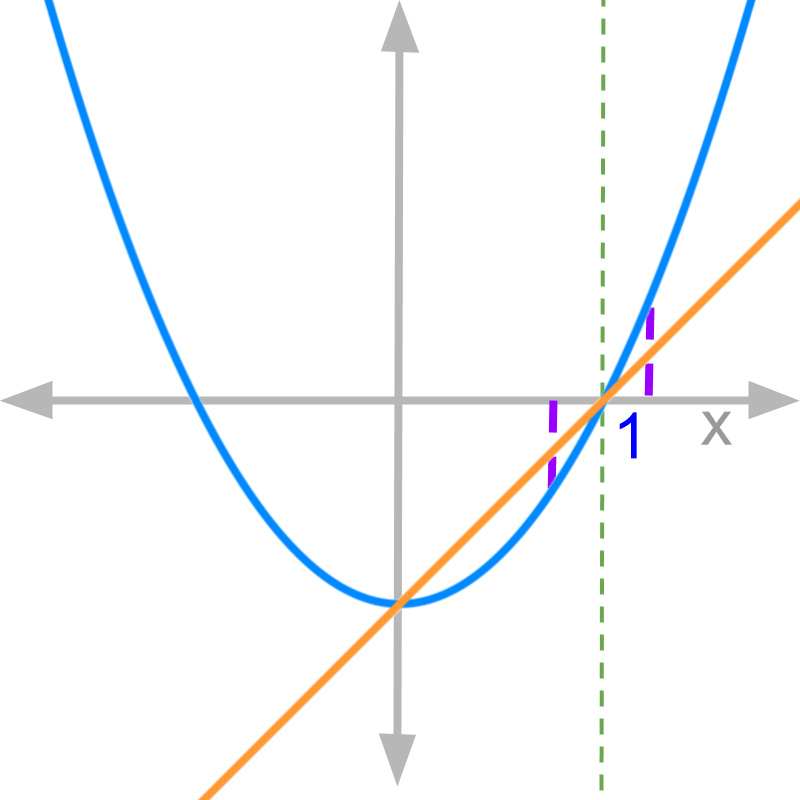maths > calculus-limits

Understanding limits with Graphs of Numerator and Denominator

what you'll learn...

Limit of Functions with graph of numerator and denominator

»  Slopes at the point x=a$x = a$ decide the limits of f(x)$f \left(x\right)$ at x=a$x = a$.→  slope of numerator at x=0$x = 0$ is 1$1$

→  slope of denominator at x=0$x = 0$ is $1$

→  Both LHL and RHL limits $= \frac{1}{1} = 1$

example

The limit of a function is computed when the function evaluates to indeterminate value $\frac{0}{0}$ at $x = a$. Seeing the division in indeterminate value $\frac{0}{0}$, the function can be given as $f \left(x\right) = \frac{\textcolor{\mathrm{de} e p s k y b l u e}{{f}_{n} \left(x\right)}}{\textcolor{c \mathmr{and} a l}{{f}_{d} \left(x\right)}}$ such that $\textcolor{\mathrm{de} e p s k y b l u e}{{f}_{n} \left(x\right)} {|}_{x = a} = 0$ and $\textcolor{c \mathmr{and} a l}{{f}_{d} \left(x\right)} {|}_{x = a} = 0$.Consider the function $f \left(x\right) = \frac{\textcolor{\mathrm{de} e p s k y b l u e}{{x}^{2} - 1}}{\textcolor{c \mathmr{and} a l}{x - 1}}$. The numerator and denominator evaluate to $0$ at $x = 1$. The figure plots the numerator and denominator : numerator is the curve in blue, and denominator is the line in orange.Given the graphs of numerator and denominator of the function $f \left(x\right) = \frac{\textcolor{\mathrm{de} e p s k y b l u e}{{x}^{2} - 1}}{\textcolor{c \mathmr{and} a l}{x - 1}}$. The vertical purple lines, show $1 - \delta$ and $1 + \delta$.

The values of denominator at $1 - \delta$ and $1 + \delta$ are $- \delta$ and $+ \delta$ respectively.

The values of numerator at $1 - \delta$ and $1 + \delta$ are $- 2 \delta + {\delta}^{2}$ and $+ 2 \delta + {\delta}^{2}$ respectively.

Given the graphs of numerator and denominator of the function $f \left(x\right) = \frac{\textcolor{\mathrm{de} e p s k y b l u e}{{x}^{2} - 1}}{\textcolor{c \mathmr{and} a l}{x - 1}}$. The vertical purple lines, show $1 - \delta$ and $1 + \delta$.

It is noted that the slope of the line defines the values of numerator at $1 - \delta$ and $1 + \delta$.

formal restatement

Given the function $f \left(x\right) = \frac{\textcolor{\mathrm{de} e p s k y b l u e}{{f}_{n} \left(x\right)}}{\textcolor{c \mathmr{and} a l}{{f}_{d} \left(x\right)}}$ such that $\textcolor{\mathrm{de} e p s k y b l u e}{{f}_{n} \left(x\right)}$${|}_{x = a} = 0$ and $\textcolor{c \mathmr{and} a l}{{f}_{d} \left(x\right)} {|}_{x = a} = 0$.

$f \left(x\right) {|}_{x = a + \delta}$
$\quad \quad = \textcolor{\mathrm{de} e p s k y b l u e}{{f}_{n} \left(x\right)} {|}_{x = a + \delta} \div \textcolor{c \mathmr{and} a l}{{f}_{d} \left(x\right)} {|}_{x = a + \delta}$
$\quad \quad = \left[\textcolor{\mathrm{de} e p s k y b l u e}{{f}_{n} \left(x\right)} {|}_{x = a + \delta} - {f}_{n} \left(a\right)\right]$
$\quad \quad \quad \quad \div \left[\textcolor{c \mathmr{and} a l}{{f}_{d} \left(x\right)} {|}_{x = a + \delta} - {f}_{d} \left(a\right)\right]$
as ${f}_{n} \left(a\right) = 0$ and ${f}_{d} \left(a\right) = 0$.

$\quad \quad = \frac{\textcolor{\mathrm{de} e p s k y b l u e}{{f}_{n} \left(x\right)} {|}_{x = a + \delta} - {f}_{n} \left(a\right)}{\delta}$
$\quad \quad \quad \quad \div \frac{\textcolor{c \mathmr{and} a l}{{f}_{d} \left(x\right)} {|}_{x = a + \delta} - {f}_{d} \left(a\right)}{\delta}$
$\quad \quad = \textcolor{\mathrm{de} e p s k y b l u e}{\textrm{s l o p e} {f}_{n} \left(x\right) {|}_{x = a}} \div \textcolor{c \mathmr{and} a l}{\textrm{s l o p e} {f}_{d} \left(x\right) {|}_{x = a}}$.

summary

Geometrical representation of Limits: If $f \left(x\right) = \frac{\textcolor{\mathrm{de} e p s k y b l u e}{{f}_{n} \left(x\right)}}{\textcolor{c \mathmr{and} a l}{{f}_{d} \left(x\right)}}$, where
$f \left(x\right) {|}_{x = a} = \frac{0}{0}$;
$\textcolor{\mathrm{de} e p s k y b l u e}{{f}_{n} \left(x\right)} {|}_{x = a} = 0$ and
$\textcolor{c \mathmr{and} a l}{{f}_{d} \left(x\right)} {|}_{x = a} = 0$, then

•  the slopes on the left of $x = a$ define the left-hand-limit and

•  the slopes on the right of $x = a$ define the right-hand-limit.

The slopes referred are for the numerator and denominator.

Outline